# Project management and scheduling, mathematical theory of

Jump to: navigation, search

The mathematical description and methods for solving a special class of mathematical programming problems, formulated in terms of optimal resource allocation on graphs and networks.

## Basic terminology.

A project is a set of interrelated tasks, or operations, that must be performed in a certain order so as to reach some goal. Events are starting and finishing points of various operations of the project.

The operations of the project are interrelated through precedence relations of two types, NOT BEFORE and NOT LATER. Let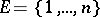be a given set of events of the project, and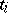the (unknown) time at which the event,, occurs. The NOT BEFORE relations denote that a certain operation can be started (and/or can be finished) not before than in, the given amount of time units, after some other operation has started (and/or finished):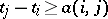,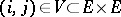.

The NOT LATER relations denote that some operation is to be started (or, equally possible, to be finished) not later than the given time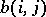before some other operation will start (finish):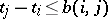,.

One of the traditional graphical forms of project representation is a directed graph, or a network, in which arcs are identified with project operations and nodes with events (cf. Network model). The graph contains, also, additional arcs in order to properly represent the precedence relations between operations.

The resulting graphhaving node setand arc setis called a PERT (for Project Evaluation and Review Technique), or CPM (Critical Path Method) network [a1], [a2].

## Problem statement.

The mathematical theory of project management and scheduling seems to have begun with the solution of the following problem [a2].

### Problem 1

(the critical path problem in acyclic networks). Letbe a PERT network, with node setand arc set, representing a project. For some arcs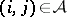, let there be given, their non-negative lengths, corresponding to the directions of project operations. Given two fixed nodes, the startand the finish, the problem is to find the longest directed path fromto(called the "critical path" ). The critical path determines the shortest possible time in which the entire project portrayed bycan be completed.

It is not necessary for a PERT network to be acyclic. The dynamic programming technique that solves efficiently Problem 1, also solves successfully the following problem in networks with cycles [a3], [a4].

### Problem 2

(PERT-TIME problem in general networks).

Let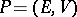be a project with event setand operation set; precedence relations of two types, NOT BEFORE and NOT LATER, are imposed on the operations of:(a1)

The PERT network, corresponding to the project, is constructed as follows: its node set is identified with the event set of; its arc setis defined as, where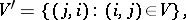with the arc lengths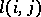being defined as follows: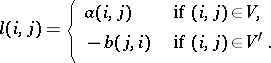Given two fixed nodes, the startand the finish, the problem is to find the shortest possible time in which the projectcan be completed (the latter being equal to the length of the longest path fromto). More sophisticated formulations of project management and scheduling problems take into consideration costs of operations [a5], [a6].

### Problem 3

(PERT-COST problem in acyclic networks).

Letbe a project with event setand operation set. Only one type of precedence relation, NOT BEFORE, is presumed to be imposed on operations in this model. The corresponding PERT network is assumed to be acyclic.

Associated with each operation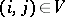are its "normal" completion time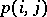, its "crash" completion timeand the costof shortening the operation by one time unit. Denoting by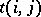the actual (unknown) duration of the operation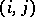, the latter quantity is to be between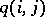and,, and the cost of the operationis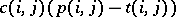. The problem is to find the minimal cost of shortening the project to a given duration.

### Problem 4

(parametric PERT-COST) [a5], [a6]. This is the same as Problem 3, except that the minimal cost of the entire project is to be determined for each feasible project duration time.

### Problem 5

(just-in-time PERT-COST in general networks) [a7]. This is an extension of Problem 3. Letbe a project with two types of imposed precedence relations, NOT BEFORE and NOT LATER, the same as described by (a1) in Problem 2. In particular, the constraints (a1) may include the requirement that certain crucial events of the project occur just in time.

The corresponding PERT network may have arc lengths of arbitrary sign and may contain cycles (necessarily, of non-positive total length).

Let the event setbe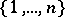. Assume the problem's objective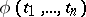to be the sum of piecewise-linear convex non-monotone functions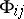,, of time shifts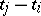, representing penalties incurred for the project operations being started/finished too early as well as too late.

The problem is to minimize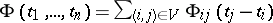subject to the network constraints (a1).

While Problems 1–5, like a number of other project management and scheduling problems, can be solved efficiently, in a polynomial number of computational steps (see, e.g., [a1][a10]), many other project management and scheduling problems have been proved to belong to the notorious class of-hard problems, which are most difficult among all the combinatorial optimization problems [a11]. Consider two typical project management and scheduling problems of the latter class.

### Problem 6

(resource-constrained PERT-TIME) [a9]. Letbe a PERT project as considered in Problems 1, 3. Supposetypes of resources are required to perform operations, each operation requiring only one type of resources, while the intensity of its consuming, in time units, being known. The durations of the operations are also known. How should the given supply of resources be assigned to the operations so that the completion time for the entire project is minimized (with the given precedence relations being taken into account)?

### Problem 7

(resource-constrained PERT-COST) [a12]. Letbe a PERT project as described in Problems 2, 5. Supposetypes of resources are required to perform operations and, for each operation, one knows a finite set of "feasible variants" of its performing, each of the variants being presented by its own set of required resources and operation duration value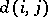. Two operations demanding the same type of resources are not permitted to occur simultaneously.

For each operationone is given: its earliest start time, latest finish time and penalties incurred if the start (the finish) of the operation takes place too early/too late.

How should the variant of performing each of the operationsand their start/finish times be chosen so that the total cost of the project, equal to the sum of penalties incurred, is minimized?

## Mathematical methods of project management and scheduling.

One of the most celebrated methods in project management and scheduling is dynamic programming. When its modification, the Bellman–Ford method of successive approximations [a10], is applied to project management and scheduling, Problem 1 is solved intime, and Problem 2 intime,being the number of nodes andthe number of arcs of the corresponding PERT network. Another important method for solving project management and scheduling problems is the network flow method. D.R. Fulkerson [a5] and J.E. Kelley [a6] employed the linear programming structure of Problem 3 and found that Problem 3 is dual to the problem of finding the minimal cost flow in a network. It is known that the Edmonds–Karp–Dinic algorithm solves the last problem in a polynomial-bounded number of computational steps [a10]. The latter fact implies the existence of polynomial procedures for solving Problems 3 and 4. Extension of the Fulkerson–Kelley approach as applied to Problem 5 is possible, leading to its polynomial-bounded solution [a7].

As for the numerical solution of the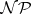-hard project management and scheduling problems (like Problems 6, 7), they have defied solution in polynomial time. Numerous computational methods suggested for solving-hard project management and scheduling problems during the last three decades (relaxation, branch-and-bound, local optimization, heuristics, etc.) at best attempted to avoid full (exponential) enumeration in practical computations, but not in the worst case. The central theoretical question here, as in integer programming in general, is whether there exist polynomial-bounded (exact) algorithms for their solution. Until now (1991) this question is still open.

The most perspective contemporary approach to practically solving-hard project management and scheduling problems is in "imbedding" sophisticated optimization procedures into the shell of a decision support system or expert system [a12].